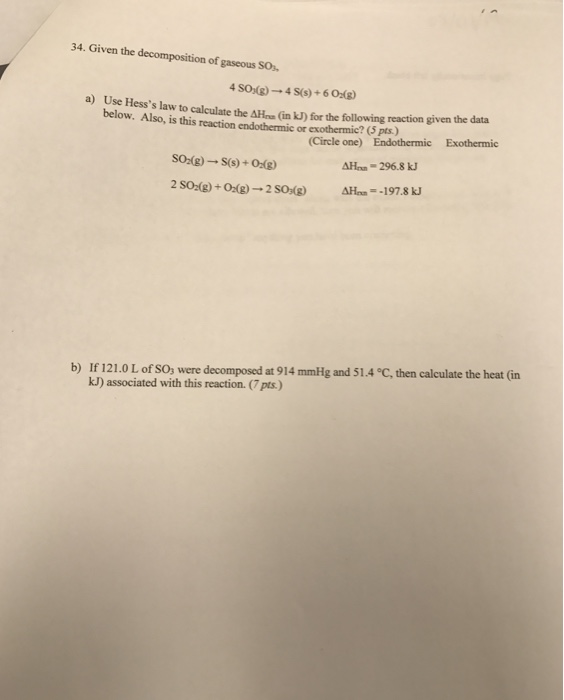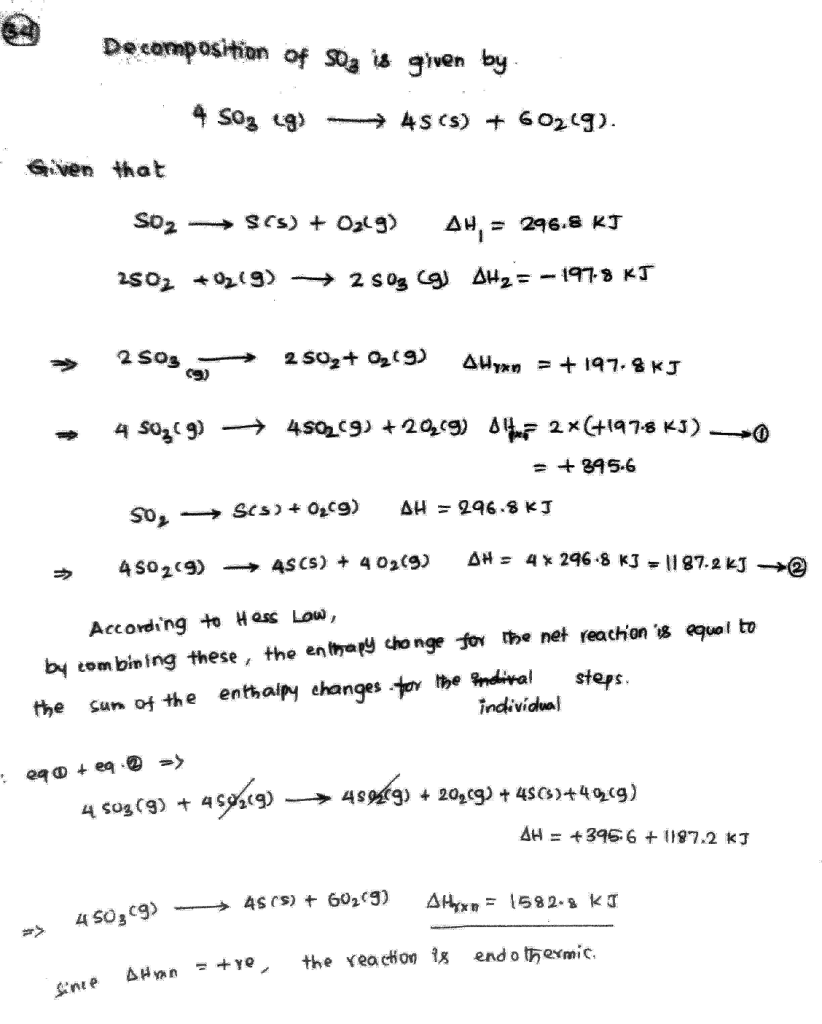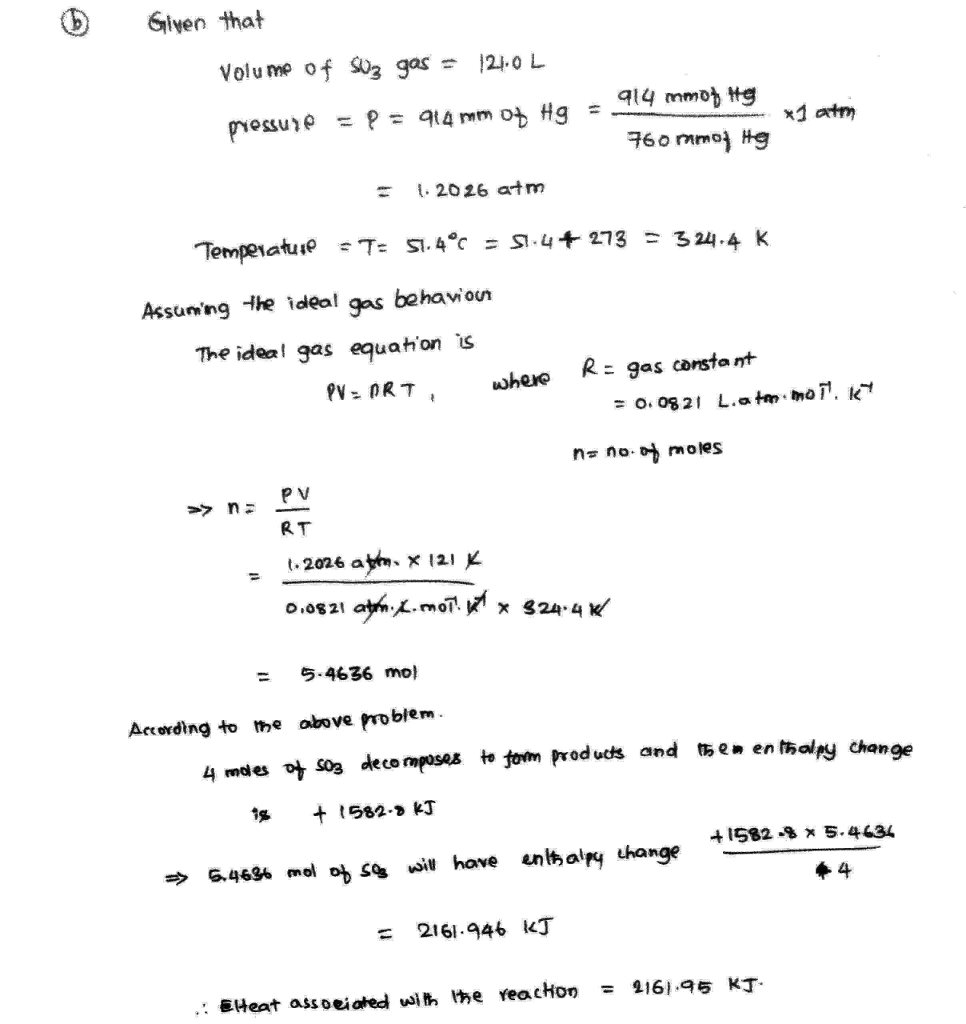# Question & Answer: Given the decomposition of gaseous SO_3, 4 SO_3(g) Rightarrow 4 S(s) + 6 O_2(g) a) Use…..Given the decomposition of gaseous SO_3, 4 SO_3(g) Rightarrow 4 S(s) + 6 O_2(g) a) Use Hess’s law to calculate the Delta H_ (in kJ) for the following reaction given the data below. Also, is this reaction endothermic or exothermic? (Circle one) Endothermic Exothermic SO_2(g) Rightarrow S(s) + O_2(g) Delta H_ = 296.8 kJ 2 SO_2(g) + O_2(g) Rightarrow 2 SO_3(g) Delta H_ = -197.8 kJ If 121.0 L of SO_3 were decomposed at 914 mmHg and 51.4 Degree C, then calculate the heat (in kJ) associated with this reaction.